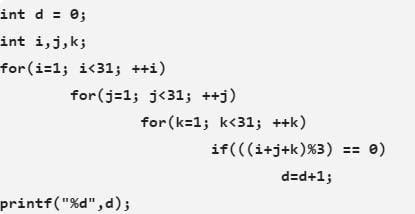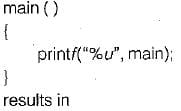# Test: Programming in C++, C & Java- 1

## 10 Questions MCQ Test GATE Computer Science Engineering(CSE) 2023 Mock Test Series | Test: Programming in C++, C & Java- 1

Description
Attempt Test: Programming in C++, C & Java- 1 | 10 questions in 30 minutes | Mock test for Computer Science Engineering (CSE) preparation | Free important questions MCQ to study GATE Computer Science Engineering(CSE) 2023 Mock Test Series for Computer Science Engineering (CSE) Exam | Download free PDF with solutions
QUESTION: 1

### Choose the correct statements:

Solution:

Enum variable type is a special flavor of a long type variable with an enum, we can specify a number of valid values for that variable and descripted constant names for the values of the enum. These values are used instead of constant. Enum variable can be declared. The advantage of enum is it provide logical clarity of a program.

QUESTION: 2

### Consider the following statements: # define hypotenuse (a, b) sqrt (a*a + b*b); The macro-call hypotenuse (a + 2, b + 3);

Solution:

The macro call will be expanded as
sqrt (a + 2 * a + 2 + b + 3 * b + 3)
i.e. sqrt (3 * a + 4 * b + 5).

QUESTION: 3

### For the previous question, which of the following macro-calls, will find the hypotenuse of a right angled triangle with sides a + 1 and b + 1 ?

Solution:

Hypotenuse of right angles triangle (a+1), (b+1) will be
h = (a + 1)2 + (b + 1)2
= a2 + 2a + 1 + b2 + 2b + 1
= a2 + b2 + 2a + 2b + 2
which is not generated by any option.

QUESTION: 4

If p is pointer to an integer and t is a pointer to a character then size of (p) will be

Solution:

Pointer to an integer and pointer to a character are equivalent when the context of size of operator is used.

QUESTION: 5

1. Both are exactly same.
2. Array is a constant pointer.
3. Pointer is an one-dimensional array.
4. Pointer is a dynamic array.

Solution:

Array and pointer are same in implementation. By using pointer we can access any of the index array. Array is a constant pointer and pointer is a one dimensional dynamic array.

QUESTION: 6

Solution:

With macro no recursion is possible, pointers cannot be used with macro identifiers and no type checking will be done and it increase the code size. So, function will be advisable to used instead of macro.

QUESTION: 7

Use of macro instead of function is recommended

Solution:

Macro will reduce the execution time and can be used when there is a loop with a function call inside.

QUESTION: 8The number of additions performed by the above program fragment is

Solution:

d = d + 1
= 9000 times
++z = 30 times
++j = 30 x 30 times
++K = 30 x 30 x 30 times
i + j + k = (30 x 30 x 30) x 2
So,
Total = 9000 + 900 + 30 + (27000 + 2 x 27000)
= 9930 + (3 x 27000)

QUESTION: 9

The following program:Solution:

Like array name, name of a function is a pointer to it. The given program results in starting address of function main (), which is pointer to it.

QUESTION: 10

Which of the following is false?

Solution:

Since address to some of the variable assigned at run time which is assigned by operating system. Two variable can have same address at different instance of time.Use Code STAYHOME200 and get INR 200 additional OFF Use Coupon Code